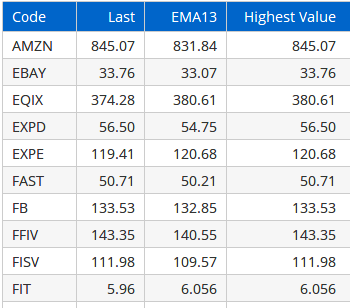Select Page

# Reply To: Custom Code MIN(a,b) formula

Optuma Forums Optuma General Discussion Custom Code MIN(a,b) formula Reply To: Custom Code MIN(a,b) formula

#28987

Hi Andy,

You can use an IF(condition, value if true, value if false)  statement for this, ie if SPX < 35 use SPX value, else use 35.

IF(CLOSE() < 35, CLOSE(), 35)

Here is an example of a watchlist column that uses the highest value between the closing value and the 13 period exponential moving average, using the following formula:

EMA13 = MA(BARS=13, STYLE=Exponential, CALC=Close);

IF(CLOSE() > EMA13, CLOSE(), EMA13)For a video on using IF statements sign in to the scripting course here and watch Lecture 5:

Intermediate Scripting